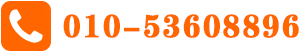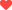# 这些基本的IGCSE数学单词你掌握了吗？

正式学习IGCSE数学课程之前，我们首先需要掌握一些基本的IGCSE数学单词，这样才能够避免学习中的一些不必要的麻烦和障碍。下面A加未来小编为大家整理了部分重点单词，来看看你是不是都掌握了呢？

Mathematics(math) is the study of numbers,quantities,shapes,and space using mathematical processes,rules,and symbols.There are many branches of mathematics and a large vocabulary associated with this subject.

Here are some math words and terms you will likely come across but may not know their precise meanings.Any word or term shown in bold is defined in the following alphabetical list of math words and terms.

Algorithm A step-by-step mathematical procedure used to find an answer.

Coefficient A number that multiplies a variable.For example,9 is the coefficient in 9x.Denominator The bottom number in a fraction.The denominator represents the number of parts into which the whole is divided.For example,6 is the denominator in the fraction.

Equation A mathematical statement used to show that two expressions are equal.It contains an equals sign.For example,16-9=7(the expression 16-9 and the expression 7 are equal).

Greatest Common Factor(greatest common divisor)The largest number that will divide two or more other numbers equally.For example,the greatest common factor of 32 and 48 is 16.

Improper Fraction(mixed fractions)A fraction that has a larger numerator than denominator.For example,is an improper fraction.

Inverse Operations Opposite or reverse operations.Addition and subtraction are inverse operations,as are multiplication and division.

Negative Number A number that is less than zero.A minus sign is used to show that a number is negative.For example,-12 is a negative number.

Numerator The top number in a fraction.The numerator represents the number of parts of the whole.For example,5 is the numerator in the fraction.

Ordinal Number A number that shows place or position,as in 2nd place.

Positive Number A number that is greater than zero.While a minus sign is used to signify a negative number,the absence of a minus sign signifies a positive number.

Prime Number A number that can be divided evenly only by itself and 1.For example,7 is a prime number.

Square Number A number that results from multiplying another number by itself.For example,49 is the square of 7(7 x 7=49).

Square Root of a Number A number that is multiplied by itself to produce a square number.For example,7 is the square root of 49.It is designated by the symbol√.

Variable A quantity that can change or vary,taking on different values.It is typically represented by a letter of the alphabet.For example x is the variable in 9x(x can be any number that is being multiplied by 9).

怎么样，这些IGCSE数学单词你都掌握了吗？这些只是在数学中发现的许多单词和术语中的一部分。在你学习数学的过程中，了解你将遇到的单词和术语的含义是很重要的。所以大家一定要做好单词的积累和掌握哦！喜欢 

## 最新文章 More## 相关推荐#### IGCSE商务研究考什么？

IGCSE商务研究考什么？.....

## 猜你喜欢 More